HOME COURSES PREVIEW REVIEW ABOUT CONTACTToll-Free Info & Ordering M-F: 9am-5pm (PST): (877) RAPID-1024/7 Technical SupportQUICK TOURMember Login:Rapid Courses Catalog Mathematics in 24 Hours Chemistry in 24 Hours Biology in 24 Hours Physics in 24 HoursMath Survival Weekly
Get the insider's tips and tricks in how to survive your math course and ace the next test. Subscribe the Web's only math weekly newsletter for students and learn:
- How to Study Math Effectively
- How to Take Math Courses Strategically
- How to Solve Math Problems Systematically
- How to Score High in Math Exams
- How to Master Math Rapidly

Enter your name and email below and get started today!Math Study Lounge These study sheets are for quick review on the subjects. Refer to our rapid courses for comprehensive review.     - Getting Started with Algebra     - Geometry Basics     - How to Solve Math Problems     - Trigonometry Quick Review     - Statistics At-A-Glance     - Calculus PreviewHome » High School Algebra 2

Counting and Probability

 Topic Review on "Title": Fundamental Counting Principle: Suppose two operations are to be performed in order: With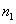possible outcomes for the first, and for each of these there are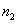possible outcomes for the second. Hence, the total number of possible outcomes is given by the product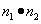. Permutations: A permutation is an ordered arrangement of a set of objects in a row. Combinations: For any natural number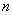, we define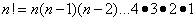. Suppose r objects are selected from a set of n objects without regard to order, each such selection is called a combination, denoted by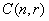or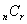.  Theorem of Combination: The number of combinations taken r at a time of a set of n objects is given by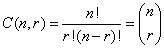. Probability: The probability of any event H is the sum of the probabilities of those outcomes of the sample space which belongs to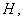denoted by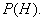Probability of Successes for H:Theorem I of Overlapping Events: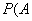or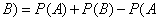and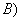Theorem II of Disjoint Events: If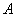and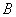are mutually exclusive events, thenor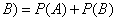. Theorem of Dependent Events: If the probability of an event A depends on the occurrence of an event B, thenand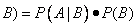wherethe probability that ifhas occurs, thenoccurs. Theorem of Independent Events: If A and B are independent events thenand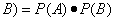.

Rapid Study Kit for "Title":
 Flash Movie Flash Game Flash Card Core Concept Tutorial Problem Solving Drill Review Cheat Sheet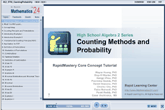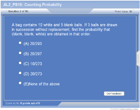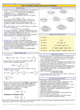"Title" Tutorial Summary : This tutorial shows probability and counting and their important concepts. Tree diagrams and illustrations are used to show permutations in the tutorial examples. An example presents the Fundamental Counting Principle. Factorials and tree diagrams are use to show combinations in the tutorial examples. The theorem of combination is presented in one of the examples to introduce the different probability distributions. The probability distributions are described in these examples. The graphical representation and tree diagrams are used to define probability and its use. The probability theorems are introduced with problems where they can be utilized. The probabilities of disjoint and overlapping events are presented in some of the tutorial examples.

 Tutorial Features: Specific Tutorial Features: • Several example problems with step by step illustrations of solutions. • Tree diagrams are used to show counting and basic probabilities. • Independent and dependent events are represented graphically. Series Features: • Concept map showing inter-connections of new concepts in this tutorial and those previously introduced. • Definition slides introduce terms as they are needed. • Visual representation of concepts • Animated examples—worked out step by step • A concise summary is given at the conclusion of the tutorial.

 "Title" Topic List: `Counting Principle and Permutations Fundamental Counting Principle Permutations and their definitionsCombinations Factorial Notation Combinations and their definitionsBinomial Distributions and the Binomial TheoremThe definition of probability and its use in applications problems Probability TheoremsThe probability of disjoint and overlapping Events Overlapping Events Theorem Disjoint Events TheoremProbability of Independent and Dependent Events`

See all 24 lessons in High School Algebra 2, including concept tutorials, problem drills and cheat sheets: Teach Yourself High School Algebra 2 Visually in 24 Hours

 ©2014 Rapid Learning Center. Privacy Policy | Disclaimer Chemistry Survival Publishing, Biology Survival Publishing and Physics Survival Publishing are the divisions of Rapid Learning Inc.